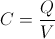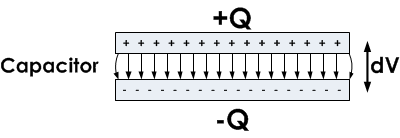# Capacitance

## What is capacitance?

Electric capacitance is the ability of a conducting body to accumulate charge. The capacitance value of a capacitor is obtained by using the formula:where C is the capacitance, Q is the amount of charge stored on each electrode, and V is the voltage between the two electrodes. In real life circuits the amount of charge on one plate equals the amount of charge on the other plate of a capacitor, but these two charges are of different signs. By examining this formula it can be deduced that a 1 F capacitor holds 1 C of charge when a voltage of 1V is applied across its two terminals.## The unit of capacitance

The unit of capacitance is a Farad [F]. This unit can be somewhat impractical. From the vantage point of most electrical engineers, one farad is a huge capacitance value. Most electronic circuits use capacitors of only up to a few mF. There are several good reasons for this.

One reason is that, when dealing with signals in an electrical circuit, as the frequency of the signal increases, the need for high capacitance capacitors decreases because, at higher frequencies, even a small capacitor can make a big impact on the circuit. Since most modern digital circuitry has a tendency to move towards higher frequencies in order to meet demands for improved processing speed, these circuits mostly use capacitors of only up to a few mF. As a result, the need for large capacitors is virtually non-existent in the signals processing parts of electrical circuits.

Another reason is that high capacitance capacitors are physically large. Therefore, the use of such capacitors is avoided, especially in mobile devices. However, there have been recent technology advances in the field of supercapacitors. Thanks to these advances, it is now possible to manufacture capacitors with capacitance values as high as several thousand farads which are comparable in size to a beverage can. These supercapacitors are under development to replace batteries in various applications, such as electric cars or even cell phones if scientists manage to reduce their size to an acceptable degree. A smaller 1F supercapacitor can be manufactured in a size comparable to a size of a few dimes stacked on top of each other.

## Capacitance characteristics of real capacitors

An ideal capacitor has a fixed capacitance value. However, the capacitance of a real capacitor can change due to several reasons. In most cases, the dielectric used in the capacitor is not ideal and the dielectric constant can be affected by certain factors.

Voltage applied to the capacitor can change the dielectric constant of the dielectric. This change directly influences the capacitance of such a capacitor. For example, this effect is pronounced when ferroelectric materials are used as the dielectric. Such an effect can be useful in some instances, and there are special devices, such as the varicap diode, which take advantage of voltage-dependent capacitance in a circuit. The name varicap comes from words VARIable CAPacitance. These diodes are used as voltage-controlled capacitors and are sometimes used in radio and TV tuners, phase-locked loops and amplifiers, as well as other circuits.

The frequency of the signal present at the capacitor's terminals can also affect its capacitance. This effect is called dielectric dispersion, and happens because the polarization of the dielectric lags behind the rapidly changing signal. At low signal frequencies this effect is negligible, however at high frequencies this effect can be quite pronounced. An example application where frequency-dependent capacitance variations have to be taken into account are MOS transistors, whose gate capacitance varies with frequency.

The age of a capacitor also has an influence on its capacitance. Some capacitors are more stable over time, while others have a relatively short life due to aging effects. For example, the electrolyte in an electrolytic capacitor may dry out over years, even when it's not used in a circuit. As time passes, the capacitance changes from the designed value and such a change could eventually lead to a circuit malfunction. For this reason, some capacitors have a defined estimated shelf life as well as an estimated life in a circuit.

## Stray capacitance

While capacitors are designed to deliberately introduce a certain capacitance into a circuit, in some other devices such as resistors, inductors or even ordinary wires, capacitance is considered an unwanted, parasitic effect. This is especially important at high frequencies, where such capacitances can make a large impact on the operation of a circuit. If two signal lines in an integrated circuits run adjacent to each other, stray capacitance can introduce a considerable amount of noise on one line if another line is driven with a high frequency signal, for example a 3.2GHz clock. This is called cross-talk and can pose a serious problem for integrated circuit engineers. The longer the two lines run adjacent to each other and the shorter the distance between them, the more pronounced this effect is. To counteract this problem, the circuit designer must maintain sufficient separation between such lines. In sensitive high frequency circuits such as oscillators and filters, even small parasitic capacitances of devices as simple as resistors must be considered during the design process. Parasitic capacitances can never be completely eliminated, but they can be reduced by using short leads, preferably SMD technolgy and devices specially designed to reduce their capacitance, such as low-capacitance resistors. For example, if a microcontroller uses a crystal oscillator, the rule of thumb is to place the crystal and all supporting elements physically as close to the microcontroller as possible, to reduce capacitance generated by the wires on the PCB (printed circuit board) and thereby the noise which results from crosstalk.

1 Comment•Md Taniqur Rahman March 04, 2021

Thanks for the information

Like.